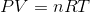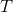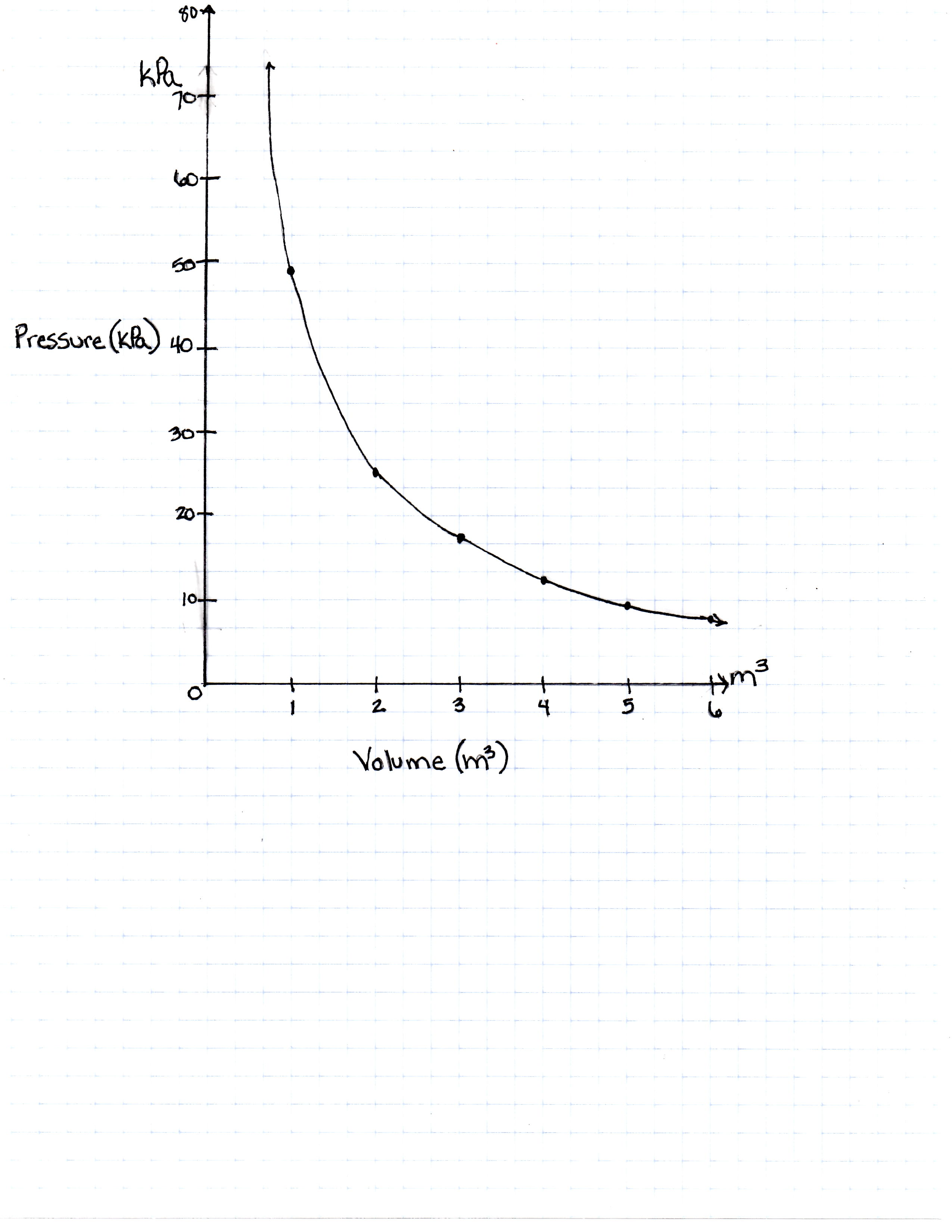Test: ACT Science

The Ideal Gas Law is as follows:is pressure as measured in Pascals,is volume as measured in cubic meters,is the number of moles of the gas,is the gas constant known as 8.314 Joules per mole times Kelvin, andis the temperature measured in Kelvin.

A class of students began studying the Ideal Gas Law and how the Pressure and the Volume relate to one another.  They took 20 moles of a sample gas and kept the room at a temperature of 300 Kelvin.  They then used different sized containers of the gas to limit and expand the volume.  At each different volume, they measure the pressure of the gas on its container.  The table they made from their results is seen in table 1.

 Volume of the container Pressure Measured in Pascals 1 cubic meter 49,884 Pascals 2 cubic meters 24,942 Pascals 3 cubic meters 16,628 Pascals 4 cubic meters 12,471 Pascals 5 cubic meters 9,976.8 Pascals 6 cubic meters 8,314 Pascals 7 cubic meters 7,126.2 Pascals

TABLE 1

And they graph their findings in Figure 1.FIGURE 1

 1 If the Kelvin temperature were to rise, what would happen to the pressure?

The temperature has no predictable effect on the pressure because there are too many other variables

Pressure increases but only until Kelvin reaches 500, and then it decreases

Pressure remains constant

Pressure increases

Pressure decreases

1/15 questions

0%

Access results and powerful study features!

Take 15 seconds to create an account.